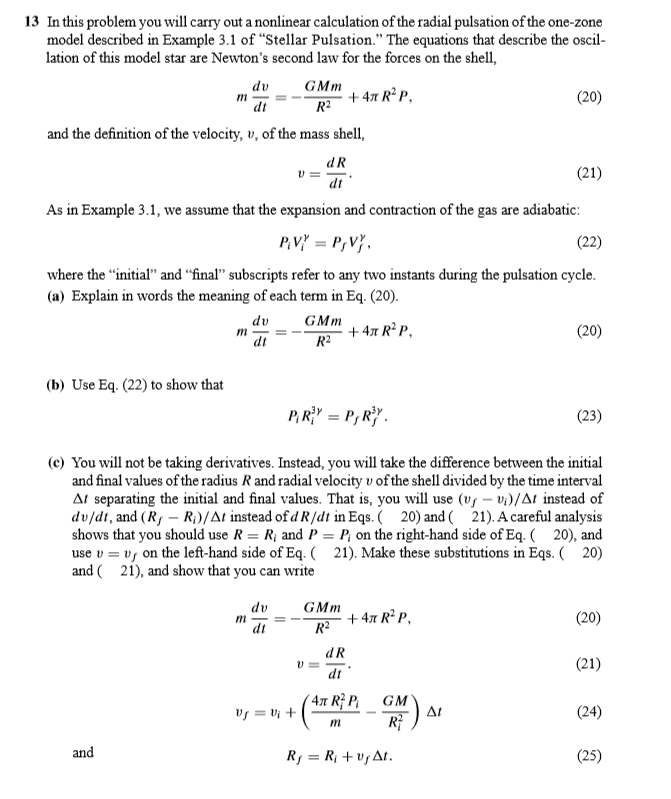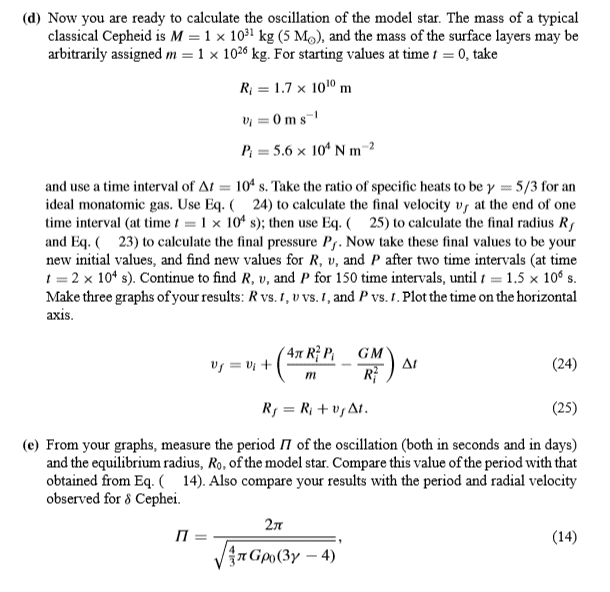# Numerically simulating stellar pulsation using a "one zone" model

Homework Statement:
Write a code that will simulate stellar pulsation using a "one zone" model, and plot various graphs
Relevant Equations:
The relevant equations are given in the image attached
The question is taken from An Introduction to Modern Astrophysics by Carroll and Ostlie. I did manage to do the entire question and plot the relevant graphs but I just wanna to investigate a bit more. For example I wanna look at how the graph would like in the case of the Sun. I don't know what the right value for pressure I would need to input in order to get the right graph, with the right amplitude and period. The value for P in part d of the question seems arbitrary to me.•PhDeezNutz